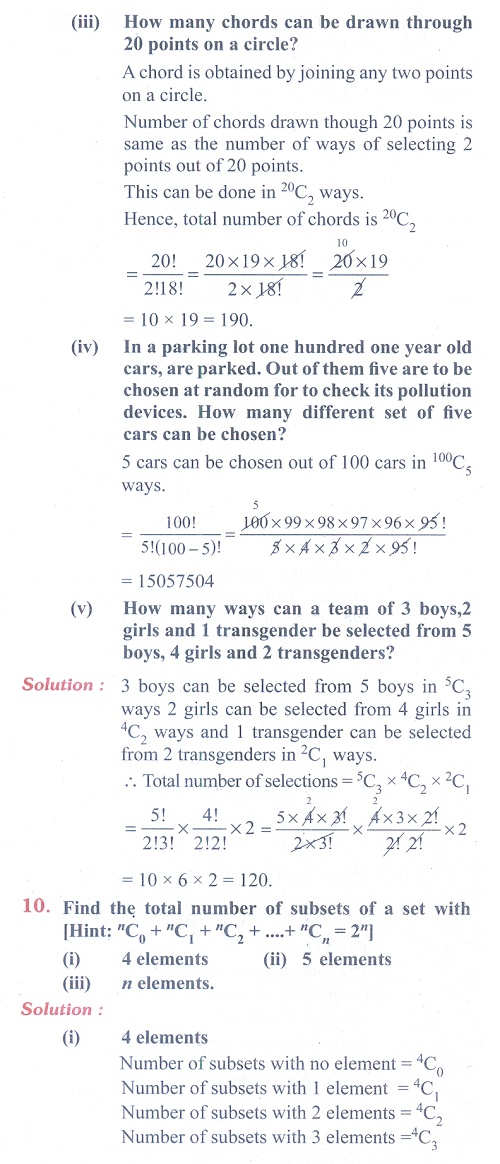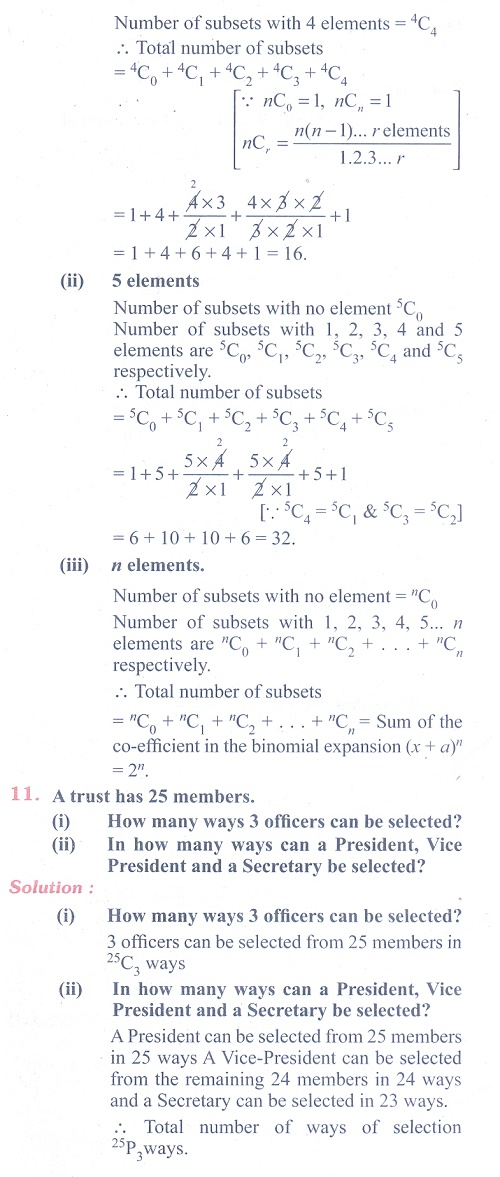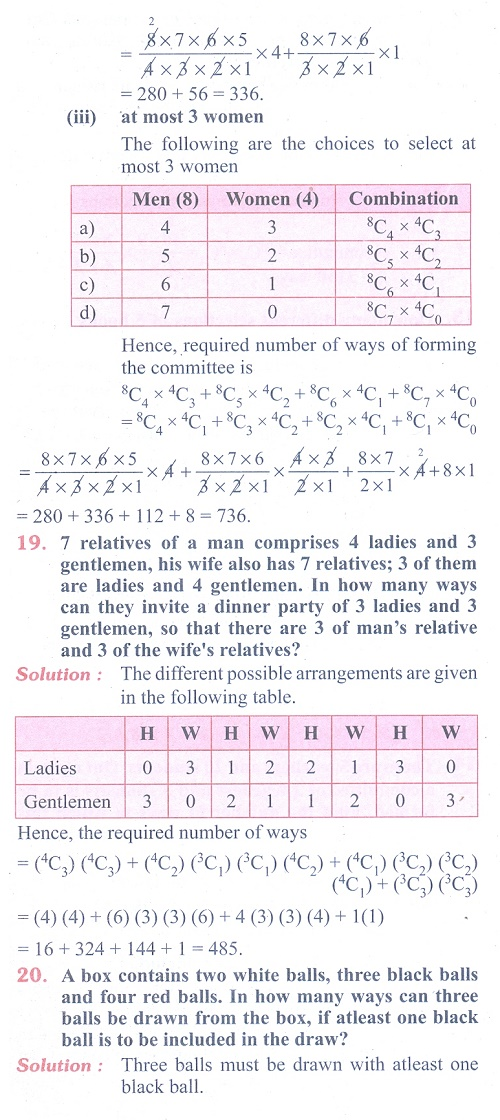Home | | Maths 11th std | Exercise 4.3: Combinations

# Exercise 4.3: Combinations

Maths Book back answers and solution for Exercise questions - Mathematics : Combinatorics and Mathematical Induction: CombinationsTags : Problem Questions with Answer, Solution | Mathematics , 11th Mathematics : UNIT 4 : Combinatorics and Mathematical Induction
Study Material, Lecturing Notes, Assignment, Reference, Wiki description explanation, brief detail
11th Mathematics : UNIT 4 : Combinatorics and Mathematical Induction : Exercise 4.3: Combinations | Problem Questions with Answer, Solution | Mathematics